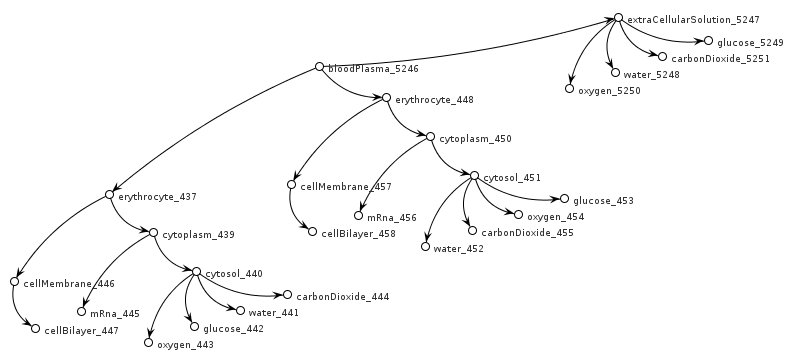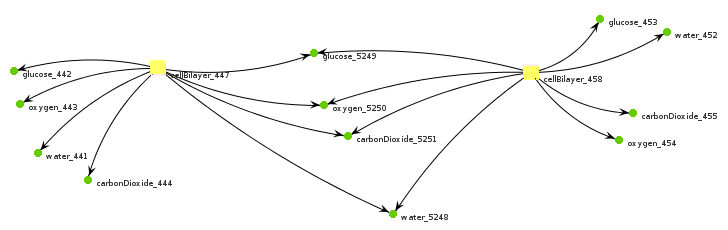# The Azimuth Project Experiments in bigraphs (Rev #4, changes)

Showing changes from revision #3 to #4: Added | Removed | Changed

The following wikipedia definition of bigraph is probably impossible to understand unless you already know what it’s talking about.

A bigraph is a 5-tuple:

$\left(V,E,\mathrm{ctrl},\mathrm{prnt},\mathrm{link}\right):⟨k,X⟩\to ⟨m,Y⟩$

where $V$ is a set of nodes, $E$ is a set of edges, $\mathrm{ctrl}$ is the control map that assigns controls to nodes, $\mathrm{prnt}$ is the parent map that defines the nesting of nodes, and $\mathrm{link}$ is the link map that defines the link structure.

The notation $⟨k,X⟩\to ⟨m,Y⟩$ indicates that the bigraph has $k$ holes (sites) and a set of inner names $X$ and $m$ regions, with a set of outer names $Y$. These are respectively known as the inner and outer interfaces of the bigraph.

I’ve starting reading the bigraph literature, and I think I understand a bit more about it now. I’m a software developer with a background in the Real-time Object-Oriented Modeling (ROOM) approach, and the author of a tool called Xholon based on ideas from ROOM. I claim that bigraphs are analogous to ROOM models. In the rest of this article, I present several diagrams that were generated by a Xholon model on cell biology. I informally describe each diagram using the vocabulary from the wikipedia article on bigraphs. I’m not an expert on bigraphs, so this is just my understanding based on my own background. The first diagram below represents a bigraph.

V: Each rounded rectangle in the diagram is a node. There are 26 nodes in the set V (where V stands for vertex).

E: Each thin green line in the diagram is an edge. There are 16 edges in the set E. The small black circles where edges meet nodes are called ports in the bigraph (and ROOM) literature but are not mentioned in the part of the wikipedia description that’s quoted above.

ctrl: Each node in the diagram is classified by a control, which is the name of the node’s class. The bigraph literature mentions various ways of classifying nodes - by sort or type, or by its signature which has to do with how many ports it has. In the diagram, BloodPlasma, Erythrocyte, CellBilayer, and Glucose are some of the 12 different controls (or classes using object-oriented software development terminology). Each node is mapped to a control (class).

prnt: The nodes in the diagram are nested inside each other. Each node has a parent, except for the top-level BloodPlasma node. So each node is mapped to a parent, for example: Erythrocyte is mapped to its parent BloodPlasma, and each of the two CellBilayer nodes is mapped to a different CellMembrane node.

link: Some of the nodes in the diagram are linked by edges. For example, each of the CellBilayer nodes is linked to eight other nodes. The link map records all of the links.

The term bigraph signifies a merging of two separate graphs, the prnt graph and the link graph. It’s two, two, two graphs in one (to paraphrase an old ad jingle).

I’m not going to try to explain what k, X and m, Y part of the wikipedia explanation is. I think I understand it, but it’s mostly irrelevant for the example I’m giving here. These have mostly to do with how to compose bigger bigraphs from two or more smaller bigraphs. My example is already a complete bigger bigraph.

The diagram also includes numbers below the name of each control. These are just the number of molecules of that type at the timestep in the simulation when I captured the diagram. In terms of bigraphs, these quantities can be ignored. This is a Scalable Vector Graphics (SVG) diagram which, now that it’s been created, can be used in the simulation. Each timestep the simulation can update the quantity in the diagram. My point here is that the bigraph diagram isn’t just an abstraction, but can be used at runtime to keep track of what’s happening in the simulation.The next diagram shows only the prnt graph, as a tree structure.The next diagram shows just the link graph, as a network structure. It’s basically a Petri net, where the yellow CellBilayer nodes are transitions and the green circles are places.The last diagram is a mind map that was generated from the same Xholon simulation. I’m including it to suggest that the bigraph idea has general appeal.I’ve started a second bigraph experiment to investigate the bigraph idea of Bigraphical Reactive Systems (BRS).